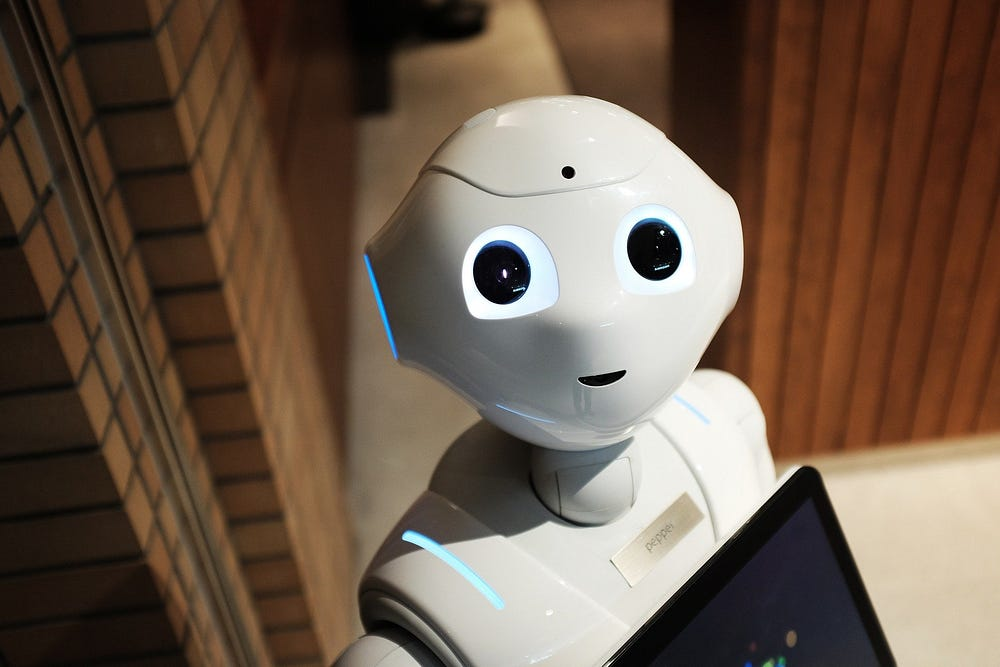Original article was published on Artificial Intelligence on Medium# Supervised Learning:

Supervised learning: a machine learning task; learning a function that maps an input to an output based on example input-output pairs

Classiﬁcation: a supervised learning task; learning a function mapping an input point to a discrete category

Nearest-neighbor classiﬁcation: an algorithm that, given an input, chooses the class of the nearest data point to that input

K-nearest-neighbor classiﬁcation: an algorithm that, given an input, selects the most common class out of the k nearest data points to that input

Weight factor: a weight given to a data point to assign it a lighter, or heavier, importance in a group

Perceptron learning rule: a method, given data point (x, y), which updates each weight according to:

`w[i] = w[i] + α(actual value - estimate) × x[i]w[i] = w[i] + α (y — h[w](x)) × x[i]`

Support vector machine: (or SVM) a popular supervised machine learning algorithm that analyzes and sorts data into two categories for classification and regression analysis

Maximum margin separator: a boundary that maximizes the distance between any of the data points

Regression: a supervised learning task; learning a function mapping an input point to a continuous value, thus being able to predict real numbered outputs

## Evaluating Hypotheses:

Loss function: a function that expresses how poorly our hypothesis performs

0–1 loss function: a simple indicator function providing information about the accuracy of predictions; returning 0 when the target and output are equal, or else it returns 1:

`L(actual, predicted) = 0 if actual = predicted,  1 otherwise`

L1 loss function: a loss function used to minimize error, by summing up all absolute differences between the true and predicted values:

`L(actual, predicted) = | actual - predicted |`

L2 loss function: a loss function used to reduce error, by summing up all squared differences between the true value and predicted values, thus penalizing single high variations:

`L(actual, predicted) = (actual — predicted)^2`

Overﬁtting: a model that fits too closely to a particular data set and therefore may fail to generalize to future data

Regularization: penalizing hypotheses that are more complex to favor simpler, more general hypotheses

`cost(h) = loss(h) + λcomplexity(h)`

Holdout cross-validation: splitting data into a training set and a test set, such that learning happens on the training set and on the test set

K-fold cross-validation: splitting data into k sets, and experimenting k times, using each set as a test set once, and using remaining data as a training set

# Reinforcement Learning:

Reinforcement learning: given a set of rewards or punishments, learn what actions to take in the future

Markov chain: a stochastic model describing a sequence of possible events in which the probability of each event depends only on the state attained in the previous event

Markov decision process: a model for decision-making, representing states, actions, and rewards

Q-learning: a method for learning a function; an estimate of the value of performing action a in state s

`Q(s, a)`

Greedy decision-making: when in state s, choose action a with max. Q(s, a)

ε-greedy: (or epsilon-greedy) a simple machine learning algorithm that takes randomness into account when deciding between explorative and exploitative options:

`1-ε (exploitative, choose estimated best move)ε (explorative, choose a random move)`